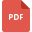# fundamental math or basic math tutorialsYear 4 Math Learning Pack
Basic Math, Grade 4, United States, Fundamental Math or Basic MathLinear and Nonlinear equations worksheet
Fundamental Math or Basic Math, Grade 8, India, Mathematical Analysis, Mathematical Logic, Linear Equations